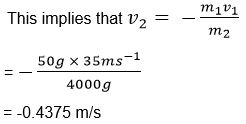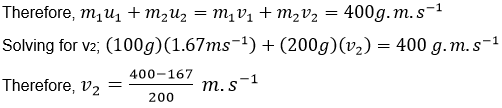• +91 9971497814
• info@interviewmaterial.com

# Chapter 9 Force And Laws Of Motion Interview Questions Answers

### Related Subjects

Question 1 : Which of the following has more inertia: (a) a rubber ball and a stone of the same size? (b) a bicycle and a train? (c) a five-rupee coin and a one-rupee coin?

Since inertia is dependent on the mass of the object, the object with the greater mass will hold greater inertia. The following objects hold greater inertia because of their mass.

Stone
Train
Five-Rupee coin

Question 2 : In the following example, try to identify the number of times the velocity of the ball changes: “A football player kicks a football to another player of his team who kicks the football towards the goal. The goalkeeper of the opposite team collects the football and kicks it towards a player of his own team”. Also identify the agent supplying the force in each case.

Let the two football teams be team A and team B respectively. Initially, the football is at rest. Now, the football is kicked by a player on team A to another player of team A. (The velocity of the ball has changed 1 time so far). This change is brought on by the force applied by the player who kicked the ball.

The football is now kicked by the other team A player towards the goal. (The velocity of the ball has changed 2 times so far). This change is also brought on due to the force applied by the team A player who kicked the ball.

The goalkeeper of team B stops the ball, bringing it to rest. (the velocity of the ball has changed 3 times so far). This change is brought on by the force applied by the goalkeeper of team B to stop the ball.

Finally, the goalkeeper of team B kicks the ball towards another player of team B. the velocity of the ball changes for a final time, bringing the total count to 4. This change is also brought on by the goalkeeper of team B, who applies the force to kick the ball forward.

Therefore, the velocity of the ball changes 4 times in this example.

Question 3 : Explain why some of the leaves may get detached from a tree if we vigorously shake its branch.

When the branch of the tree is shaken, the branch moves in a to-and-fro motion. However, the inertia of the leaves in attached to the branch resists the motion of the branch. Therefore, the leaves that are weakly attached to the branch fall off due to inertia whereas the leaves that are firmly attached to the branch remain attached.

Question 4 : Why do you fall in the forward direction when a moving bus brakes to a stop and fall backwards when it accelerates from rest?

Initially, when the bus accelerates in a forward direction from a state of rest, the passengers experience a force exerted on them in the backward direction due to their inertia opposing the forward motion.

Once the bus starts moving, the passengers are in a state of motion in the forward direction. When the brakes are applied, the bus moves towards a position of rest. Now, a force in the forward direction is applied on the passengers because their inertia resists the change in the motion of the bus. This causes the passengers to fall forwards when the brakes are applied.

Question 5 : If action is always equal to the reaction, explain how a horse can pull a cart.

When the horse walks in the forward direction (with the cart attached to it), it exerts a force in the backward direction on the Earth. An equal force in the opposite direction (forward direction) is applied on the horse by the Earth. This force moves the horse and the cart forward.

The velocity at which the horse can move by applying a force on the earth depends on the mass of the horse (and the cart attached to it). The heavier the cart, the slower the motion of the horse (for a given amount of force applied by the horse on the Earth). If the cart is too heavy, the force exerted by the horse on the Earth will be insufficient to even overcome the force of inertia. In this case, the horse will not be able to pull the cart.

Question 6 :  Explain, why is it difficult for a fireman to hold a hose, which ejects large amounts of water at a high velocity.

For the hose to eject water at high velocities, a force must be applied on the water (which is usually done with the help of a pump or a motor). Now, the water applies an equal and opposite force on the hose. For the fireman to hold this hose, he must apply a force on it to overcome the force applied on the hose by the water. The higher the quantity and velocity of the water coming out of the hose, the greater the force that must be applied by the fireman to hold it steady.

Question 7 :  From a rifle of mass 4 kg, a bullet of mass 50 g is fired with an initial velocity of 35 m s–1. Calculate the initial recoil velocity of the rifle.

Given, the Bullet’smass (m1) = 50 g

The rifle’s mass (m2)= 4kg = 4000g

Initial velocity ofthe fired bullet (v1) = 35 m/s

Let the recoilvelocity be v2.

Since the rifle wasinitially at rest, the initial momentum of the rifle = 0

Total momentum of therifle and bullet after firing = m1v1 + m2v2

As per the law ofconservation of momentum, the total momentum of the rifle and the bullet afterfiring = 0 (same as initial momentum)

Therefore, m1v1 +m2v2 = 0Therefore, the recoilvelocity of the rifle is 0.4375 meters per second in the direction opposite tothe trajectory of the bullet (backward direction).

Question 8 :

Two objects of masses 100 g and 200 g are moving along the same line anddirection with velocities of 2 ms–1 and 1 ms–1,respectively. They collide and after the collision, the first object moves at avelocity of 1.67 ms–1. Determine the velocity of the second object.

Assuming that thefirst object is object A and the second one is object B, it is given that:

Mass of A (m1)= 100g

Mass of B (m2)= 200g

Initial velocity of A(u1) = 2 m/s

Initial velocity of B(u2) = 1 m/s

Final velocity of A (v1)= 1.67 m/s

Final velocity of B (v2)=?

Total initial momentum= Initial momentum of A + initial momentum of B

= m1u1 +m2u2

= (100g) × (2m/s) +(200g) × (1m/s) = 400 g.m.sec-1

As per the law ofconservation of momentum, the total momentum before collision must be equal tothe total momentum post collision.v2 =1.165 m/s

Therefore, thevelocity of object B after the collision is 1.165 meters per second.

Question 9 : An object experiences a net zero externalunbalanced force. Is it possible for the object to be travelling with anon-zero velocity? If yes, state the conditions that must be placed on themagnitude and direction of the velocity. If no, provide a reason.

Yes, it is possible.An object moving in some direction with constant velocity will continue in itsstate of motion as long as there are no external unbalanced forces acting onit. In order to change the motion of the object, some external unbalanced forcemust act upon it.

Question 10 :

When a carpet is beaten with a stick, dust comes out of it. Explain.

Answer 10 : When the carpet is beaten with a stick, the stick exerts a force on the carpet which sets it in motion. The inertia of the dust particles residing on the carpet resists the change in the motion of the carpet. Therefore, the forward motion of the carpet exerts a backward force on the dust particles, setting them in motion in the opposite direction. This is why the dust comes out of the carpet when beaten.

Todays Deals### Chapter 9 Force And Laws Of Motion Contributorskrishan

Name:
Email:

# Latest News# 9000 interview questions in different categories